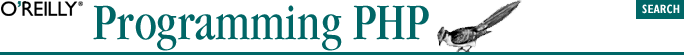home | O'Reilly's CD bookshelfs | FreeBSD | Linux | Cisco | Cisco Exam## 3.2. Defining a Function

```function [&] function_name ( [ parameter [, ... ] ] )
{
statement list
}```
```<? function column( ) { ?>
</td><td>
<? } ?>```

If you define your function with the optional ampersand before the name, the function returns a reference to the returned data rather than a copy of the data.

Let's take a look at a simple function. Example 3-1 takes two strings, concatenates them, and then returns the result (in this case, we've created a slightly slower equivalent to the concatenation operator, but bear with us for the sake of example).

#### Example 3-1. String concatenation

```function strcat(\$left, \$right) {
\$combined_string = \$left . \$right;
return \$combined_string;
}```

The function takes two arguments, \$left and \$right. Using the concatenation operator, the function creates a combined string in the variable \$combined_string. Finally, in order to cause the function to have a value when it's evaluated with our arguments, we return the value \$combined_string.

#### Example 3-2. String concatenation redux

```function strcat(\$left, \$right) {
return \$left . \$right;
}```

#### Example 3-3. Using our concatenation function

```<?php
function strcat(\$left, \$right) {
return \$left . \$right;
}

\$first = "This is a ";
\$second = " complete sentence!";

echo strcat(\$first, \$second);
?>```

This function takes in an integer, doubles it, and returns the result:

```function doubler(\$value) {
return \$value << 1;
}```

Once the function is defined, you can use it anywhere on the page. For example:

`<?= 'A pair of 13s is ' . doubler(13); ?>`
```function outer (\$a) {
function inner (\$b) {
echo "there \$b";
}
echo "\$a, hello ";
}
outer("well");